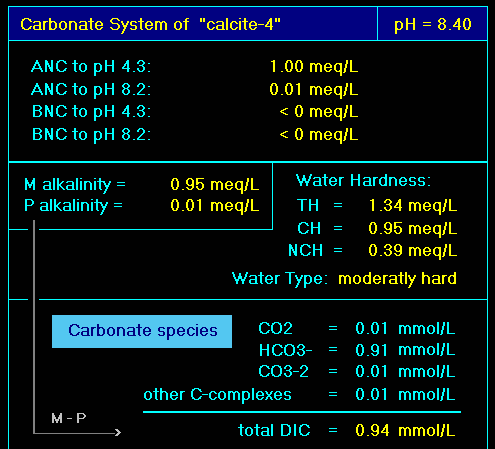# DIC as Sum of Carbonate Species

In hydrochemistry, DIC or CT is defined as the sum of all dissolved carbonate species:

 (1) DIC  =  CT  =  [CO2] + [HCO3-] + [CO3-2]

where the rectangular brackets symbolize molar concentrations. The three carbonate species are:

 •   CO2 = CO2(aq) + H2CO3 composite carbonic acid H2CO3* •   HCO3- hydrogen carbonate (bicarbonate) •   CO3-2 carbonate

DIC or CT is also abbreviated by C(4), which stands for carbon in the oxidation state IV.1 C(4) is the favored notation in hydrochemistry codes and their thdyn databases.2

Equation (1) applies only to the pure CO2-H2O-System. In real systems, additional carbonate species or complexes are present and 1 becomes:

 (2) DIC  =  CT  =  [CO2] + [HCO3-] + [CO3-2] + carbonate complexes

Typical carbonate complexes are: CaCO3(aq), CaHCO3+, MgCO3(aq), MgHCO3+, NaHCO3(aq), NaCO3-, FeCO3(aq), FeHCO3+ and others.3Carbonate Speciation

Each equilibrium calculation provides the complete carbonate speciation. One example is given in the lower part of the right screenshot. It displays the four terms of 2 that add up to DIC.

More details, can be found in the output table Ions. It contains the molar and mass concentrations of each individual carbonate complex.

Example: The Closed Carbonate System

In a closed carbonate system, the total inorganic carbon (DIC) is a conserved quantity. This is demonstrated in the stacked-area chart of a titration calculation:While each carbonate species vary with pH, their sum remains constant:

 (3) DIC  =  CO2 + HCO3- + CO3-2 + NaHCO3 + NaCO3-  =  1 mM

Footnotes

1. The counterpart of C(4) is C(-4), i.e. carbon in the oxidation state -IV. To C(-4) belong methane CH4 which predominates under strong reducing conditions.

2. C(4) represents a so-called “master species”. How it enters common thdyn databases is discussed here

3. Note the difference between the dissolved species such as CaCO3(aq) and the solid phase CaCO3(s) known as calcite. These are quite different entities.